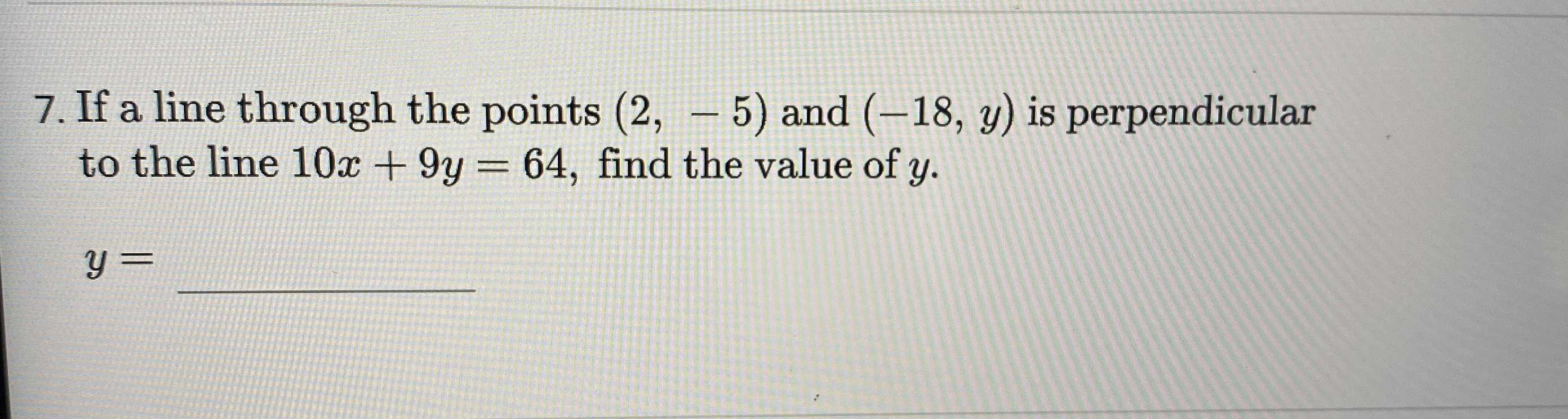### ¿Todavía tienes preguntas de matemáticas?

Pregunte a nuestros tutores expertos
Algebra
Pregunta7. If a line through the points $$( 2 , - 5 )$$ and $$( - 18 , y )$$ is perpendicular to the line $$10 x + 9 y = 64$$ , find the value of $$y$$ .

$$y =$$

$$y= -23$$